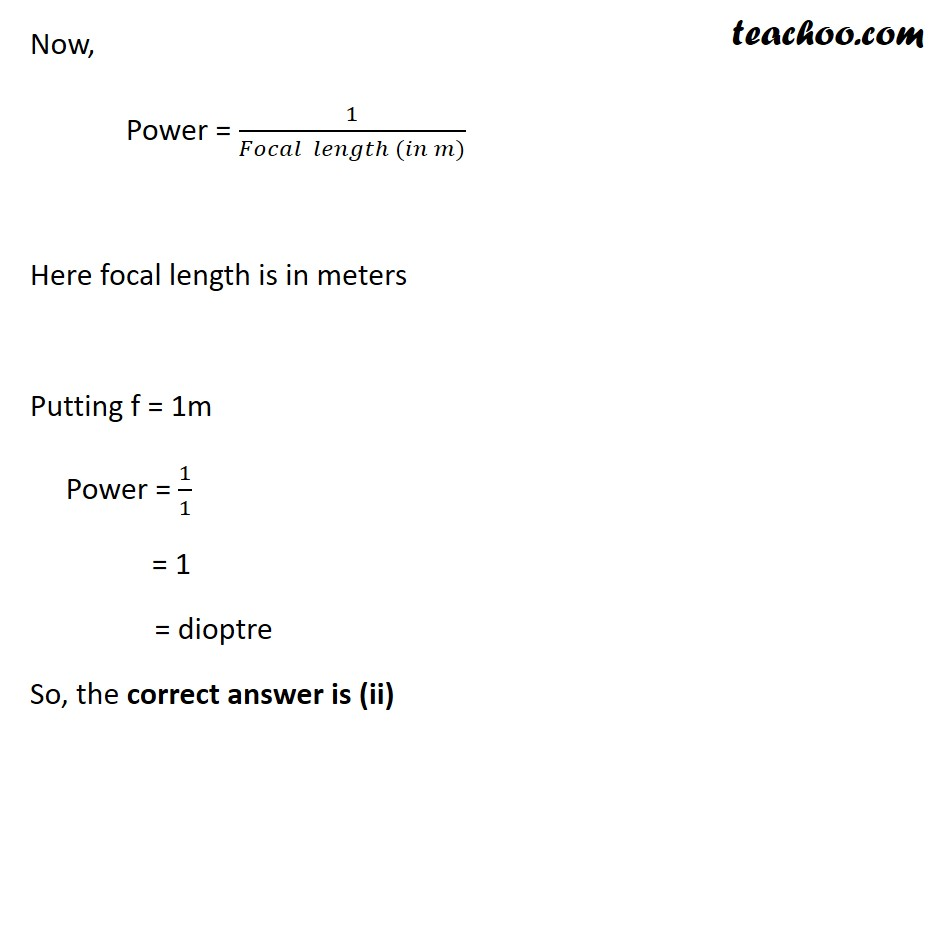Case Based Questions (MCQ)

Class 10
Chapter 10 Class 10 - Light - Reflection and Refraction

## An object is kept at a distance of 18 cm, 20 cm, 22  cm and 30 cm, from a lens of power + 5D.

### (4) 0.5 cm

Focal length of a lens = 1/Power

= ⅕ = 0.2 m

Note: focal length obtained from the power formula is always in metres .

Thus, the focal length of given lens is 20cm .

So, the correct answer is (ii)

### (iv) All the above

Since

• Focal length is 20 cm
• And focal length is positive
• It is a convex lens

Now let us go through the options.

• (i) 18cm - Object between F and P: The image produced is virtual, erect and magnified.
• (ii) 20cm -  Object at F: The image produced at F is real, inverted  and magnified.
• (iii) 22cm - Object between C and F: The image will be real, inverted and magnified.
•

Since magnified image is being formed at all object distances.

Thus, the correct answer is (iv) all the above

### (iv) At 22 and 30 cm

Ans.

As explained in question (b), for convex lens

• The object at 18 cm forms a virtual image . This cannot be captured on a screen.

• The object at 20 cm forms an image at infinity . Since the rays never meet, the image cannot be caught on a screen.

• Only the object at 22 and 30 cm form real images with rays that converge to form an image. Hence, these images can be captured on a screen.

Thus the correct answer is (iv) at 22 cm and 30 cm

### (iv) Wavelength### (iv) Concave mirror

Ans. Let us go through our options:

• A Concave lens or a convex mirror produce virtual images. These cannot be used in film projectors as images cannot be captured on a screen.
• A convex lens and concave mirror produce real, magnified images (everywhere except between F and P ) that can be captured on screen.

Learn in your speed, with individual attention - Teachoo Maths 1-on-1 Class